# 16th LSI Design Contests・in Okinawa  Design Specification - 1

## 1. Noise Cancelling Algorithm

To remove noise, there are some methods are suggested such as Microphone Array Method which is used several microphones and method using adaptive filtering to presume unknown pathway. In this simulation, the Noise Cancelling System by using Specter Subtraction Method (SS Method) which removes the noise in frequency domain is targeted.

## 1-1. Specter Subtraction Method (SS Method)

In this section, the Noise Cancelling Algorithm is explained. The algorithm removes the noise in frequency domain. A diagram of the Noise Cancelling Algorithm is shown in Figure 1.

First, the observed signal is assumed to be given by the sum of speech and noise. The observed signal, the speech and the noise at time n are defined as x(n), s(n) and d(n) respectively. Then, the observed signal x(n) is expressed by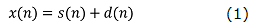when the speech and the noise are uncorrelated.

Next, to remove noise in frequency domain, the Discrete Fourier Transform (DFT) applies the observed signal x(n). After that, the observed signal specter X(l,k) from top to k in l-frame is expressed by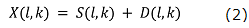when S(l,k) and D(l,k) denote the speech specter and the noise specter respectively. The estimate value of speech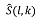is expressed by the observed signal specter X(l,k) as．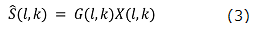Here, G(l,k) is a specter gain, and the estimate value specter is result of the observed signal specter X(l,k) multiplied by suitable specter gain G(l,k). The ideal estimate valueis expressed by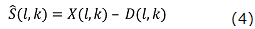By substituting eq.(4) into eq.(3), the ideal specter gain G_opt (l,k) given as．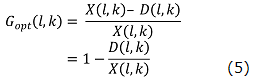Then, the speech specter X(l,k) is extracted perfectly. However, the noise specter D(l,k) can’t be obtained by only the speech specter X(l,k)’s information. Therefore, for SS Method, the noise specter estimate value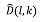can be obtained by using the ‘L’ number of frames in non-speech interval.is defined as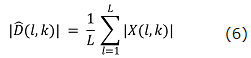The simulation of the SS method was performed by Scilab. The result is shown in Figure 2. We can download the program (here).